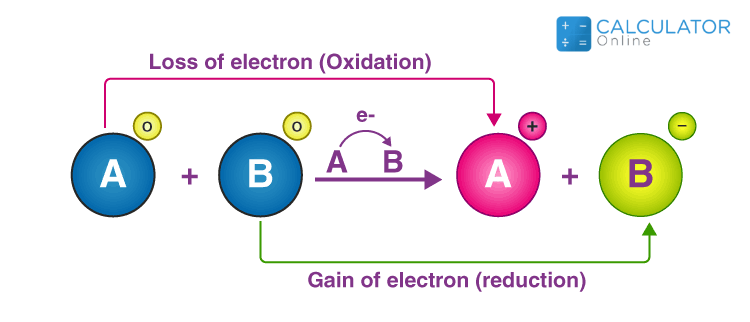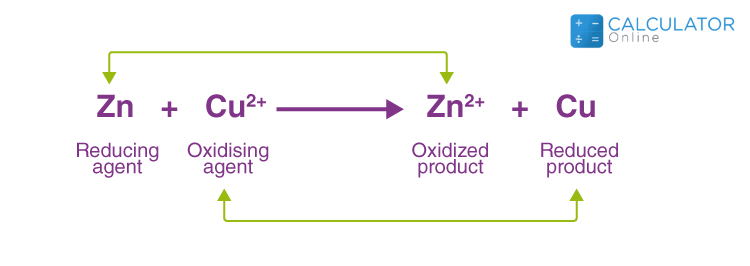•Sign In
• Blog
• Write for UsUh Oh! It seems you’re using an Ad blocker!

We always struggled to serve you with the best online calculations, thus, there's a humble request to either disable the AD blocker or go with premium plans to use the AD-Free version for calculators.

Or# Redox Reaction Calculator

Enter the chemical equation and the calculator will immediately let you know which element is oxidised and which is reduced in it.

Enter an Equation

 H He Li Be B C N O F Ne Na Mg Al Si P S Cl Ar K Ca Sc Ti V Cr Mn Fe Co Ni Cu Zn Ga Ge As Se Br Kr Rb Sr Y Zr Nb Mo Tc Ru Rh Pd Ag Cd In Sn Sb Te I Xe Cs Ba La Hf Ta W Re Os Ir Pt Au Hg TI Pb Bi Po At Rn Fr Ra Ac Rf Db Sg Bh Hs Mt Ds Rg Cn Nh FI Mc Lv Ts Og Lanthanoids Ce Pr Nd Pm Sm Eu Gd Tb Dy Ho Er Tm Yb Lu Actinoids Th Pa U Np Pu Am Cm Bk Cf Es Fm Md No Lr
 SPACE 1 2 3 4 5 6 7 8 9 0 + = CLEAR

Table of Content

 1 What is priceeight Class? 2 priceeight Class Chart: 3 How to Calculate priceeight Density (Step by Step): 4 Factors that Determine priceeight Classification: 5 What is the purpose of priceeight Class? 6 Are mentioned priceeight Classes verified by the officials? 7 Are priceeight Classes of UPS and FedEx same?

Get the Widget!

Add this calculator to your site and lets users to perform easy calculations.

Feedback

How easy was it to use our calculator? Did you face any problem, tell us!

The Redox reaction calculator recognizes both the oxidation and reduction at once, which makes us clear what is happening in the chemical reaction.This is essential when you are finding the oxidizing or the reducing power of the ions. You need to understand some of the ions are naturally oxidizing by nature and some are the reducing agents.

## What is the Redox reaction?

The Redox reaction is a type of a reaction in which both the oxidation and reduction occur at the same time.It can be difficult to figure out what is going on in the Redox reaction, especially when oxidation and reduction is happening simultaneously.The main reason for this, we aren’t able to understand where oxidation is happening and where reduction is occurring. The balancing redox reaction calculator automatically balances the chemical equation and indicates where the oxidation and reduction is happening in the chemical reaction. The Redox equation balancer automatically finds all the reducing and the oxidizing agents in the reaction.

### The reduction process:

First, we need to understand the reduction process? For students it is difficult to spot the reduction process since it is just too tedious to spot the changes.It is difficult to spot the reduction process if we are able to recognize the changes occurring in the reduction process.There basics four types of changes happening in the reduction process.

First change is the gain of electrons, the second one is the loss of oxygen. The third one is the gain of hydrogen and the fourth one is the increase of oxidation number.

The balancing redox reaction calculator exactly finds what is the reduction number of an atom. It is difficult to spot all the changes but thanks to the online redox reaction calculator that sort out the reduction process within no time.

if you are using the redox reaction calculator. Then it is just too easy to figure out where the reduction process is happening.
We can elaborate all the four changes regarded as the reduction process are shown as follows

• Gains one or more electrons
• Loses an oxygen atom or Electronegative atoms
• Gains a hydrogen atom or Electropositive atoms
• Loses and decrease in its oxidation number

## Example of the reduction process:

We are now presenting a chemical equation where the Redox reaction is happening.It is just not easy to find where the reduction is happening and where the oxidation process is happening.
2FeCl3 (aq) + H2 (g) → 2FeCl2 (aq) + 2HCl (aq)
Closely examine the chemical reaction above, here you can spot both the reduction and oxidation process.
The following changes happening in the reaction:
The FeCl3 is reduced as it is going to lose the electron negatively charged chlorine.
Hydrogen is oxidized here as it is losing the hydrogen
The redox calculator is readily spotting all the changes in the Redox reaction.

## The oxidation process:

Same like the reduction process, students do find to spot the oxidation process.It is totally a complete reversal of the reduction process. The first change in the oxidation process is the loss of electrons, and the second one is the gain of the oxygen process. The third one is the loss of the hydrogen atom, and the fourth one is the increase in its oxidation number simultaneously.The redox reaction calculator exactly finds what is the oxidation number of an atom.

We can elaborate all the four chances regarded as the reduction process are shown as follows

• Loss of one or more electrons
• Gain of an oxygen atom or Electronegative atoms
• Loss of a hydrogen atom or Electropositive atoms
• Gaining and increase in its oxidation number

## Example of the oxidation process:

Consider the reaction of Magnesium with the oxygen. In the reaction we are getting the Magnesium Oxide.
Mg(s)+ O2(g)→ 2MgO
Examine the chemical reaction above, here you can spot both the reduction and oxidation process, as we have seen in the example of the reduction process.
The following changes happening in the reaction:
The Mg is oxidized as it is going to gain the electron and it is also getting the oxygen.
Oxygen is reduced as it is losing electrons to the Mg+2 ion.
The redox calculator is readily spotting all the changes in the Redox reaction.

## Implementation of the Redox reaction:

The examples of redox reactions and the simultaneous oxidation and reduction half-reactions are indicated in the following example:
Example 1:
The Hydrogen and Fluorine Redox reaction:
Hydrogen and fluorine, the hydrogen is oxidized whereas the fluorine is reduced as it is gaining the electron and the Hydrogen is oxidized.It can be just too tedious to spot where oxidation is occurring and where the reduction is occurring,
The reaction can be written as follows.
H2 + F2 → 2HF
Oxidation half reaction: H2 → 2H+ + 2e–
Reduction half reaction: F2 + 2e– → 2F–
Both ions combine together and make Hydrogen Fluoride , we can find the balanced half reaction to find the half oxidation and reduction reaction.The oxidation reduction calculator is readily spotting the reduction and oxidizing agents. We are simply also able to find how to balance a redox reaction.
Example 2:
The reaction between Zinc and Copper
The Copper “Cu” is displaced by the Zinc “Zn” and Cu2+ is replaced by the Zinc “Zn”. The copper sulfate is converted into the Zinc sulfate.Zn (s) + CuSO4 (aq) → ZnSO4 (aq) + Cu (s)
The oxidation half-reaction: Zn → Zn2+ + 2e–
The reduction half-reaction: Cu2+ + 2e– → Cu

The redox reaction calculator is indicating the whole reaction of the reduction and oxidation.

## Types of the Redox reaction:

There are three main types of the redox reactions:

1. Decomposition reaction
2. Combination reaction
3. Displacement reaction

We try to understand all the four types of the

## 1:Decomposition reaction:

In the decomposition reaction breakdown of a compound into different compounds takes place .
The Examples of Decomposition reaction are :
2NaH → 2Na + H2
2H2O → 2H2 + O2
Na2CO3 → Na2O + CO2
The above reactions result in the disintegration of the smaller compounds in the form of
AB → A + B
But, there is a special case that confirms that all the decomposition reactions are not redox reactions. The Redox reaction calculator enables us to spot all the positives and negatives in the chemical reaction.

## 2:Combination reaction:

In the decomposition reaction reactants combine and it involve the combination of two compounds to form a compound in the following form
A + B → AB
For example:
H2 + Cl2 → 2HCl+O2→CO2
4 Fe+ 3O2→2Fe2O3
The redox equation balancer makes it clear how many electrons a substance is going to receive or lose.

## 3:Displacement reaction:

In the displacement reaction a compound is replaced by an atom or an ion of another element. We can show it as follows
X + YZ → XZ + Y
Example:
Zn (s) + CuSO4 (aq) → ZnSO4 (aq) + Cu (s)
The subcategories of the displacement reactions are as follows:

• Metal displacement Reaction
• Non-metal displacement Reaction

The balancing redox reactions calculator is swiftly spotting the metal and non-metals in the chemical reaction.

## The redox reaction calculator processing?

It can be simple and fast when you are using the balance redox reaction calculator.
Input:

• Enter the chemical equation in the calculator

Output:

When you are working with the chemical equation it can be confusing where the reducing and oxidizing agents are. You find the whole process simple when using the half reaction calculator.

• The reducing and oxidizing agents are spotted
• Gian and loss of the electron is displayed

## What are the oxidizing agents?

The oxidizing agents are the group of elements accepting the electron. It is readily reduced in an oxidation-reduction reaction.The examples of the oxidizing agents are O2,O3,H2O2, F2, HNO3 etc.

## What are reducing agents?

The reducing agents are the electron donating group of the elements.This group readily reduced the losing electrons. The examples of the oxidizing agents are Mg,Li,Br, and Fe etc.

## Are metal reducing agents?

The metals are the reducing agents as they tend to gain the electron in a chemical reaction. Like Iron, Magnesium, Sodium etc.

## Are metal oxidizing agents?

The nonmetals are the oxidizing agents as they tend to lose the electron in a chemical reaction, except hydrogen,Like Oxygen, Fluorine etc

## Conclusion:

The Redox reaction is one of the most difficult concepts to understand in chemistry. The main problem here is that students have a clear picture of the concept. This can be great to learn the Redox reactions when using the redox reaction calculator.

## References:

From the source of Wikipedia: Redox, Terminology, Oxidants

From the source of study.com: Redox-Reactions Presentation